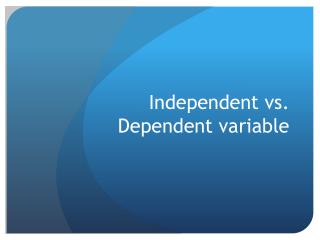DownloadDownload PresentationIndependent vs. Dependent variable

# Independent vs. Dependent variable

Download Presentation## Independent vs. Dependent variable

- - - - - - - - - - - - - - - - - - - - - - - - - - - E N D - - - - - - - - - - - - - - - - - - - - - - - - - - -
##### Presentation Transcript

1. Independent vs. Dependent variable

2. Variables • In scientific experiments there are two kinds variables: 1) the independent variable and 2) the dependent variable • It is important to be able to identify which is which in an experiment. • What is the difference between a dependent variable and an independent variable?

3. Independent Variable • An independent variable is exactly what it sounds like. It is a variable that stands alone and isn't changed by the other variables you are trying to measure. • For example, someone's age might be an independent variable. Other factors (such as what they eat, how much they go to school, how much television they watch) aren't going to change a person's age.

4. Dependent Variable • Just like an independent variable, a dependent variable is exactly what it sounds like. It is something that depends on other factors

5. Dependent Variable • For example, a test score could be a dependent variable because it could change depending on several factors such as how much you studied, how much sleep you got the night before you took the test, or even how hungry you were when you took it.

6. Independent Vs. Dependent Variable • When you are looking for some kind of relationship between variables you are trying to see if the independent variable causes some kind of change in the other variables, or dependent variables. • Usually when you are looking for a relationship between two things you are trying to find out what makes the dependent variable change the way it does.

7. Independent Vs. Dependent Variable • Many people have trouble remembering which is the independent variable and which is the dependent variable. • An easy way to remember is to insert the names of the two variables you are using in this sentence in they way that makes the most sense.

8. Independent Vs. Dependent Variable • Then you can figure out which is the independent variable and which is the dependent variable: (Independent variable) causes a change in (Dependent Variable) and it isn't possible that (Dependent Variable) could cause a change in (Independent Variable).

9. Independent Vs. Dependent Variable • For example: (Time Spent Studying) causes a change in (Test Score) and it isn't possible that (Test Score) could cause a change in (Time Spent Studying). • We see that "Time Spent Studying" must be the independent variable and "Test Score" must be the dependent variable because the sentence doesn't make sense the other way around.

10. Graphing Independent & Dependent Variable • When we graph it’s important to put the independent variable and the dependent variable on very specific axes. • The independent variable is always on the x-axis • The dependent variable is always on the y –axis

11. Graphing Independent & Dependent Variable • When we graph it’s important to put the independent variable and the dependent variable on very specific axes. • The independent variable is always on the x-axis • The dependent variable is always on the y –axis

12. Graphing Independent & Dependent Variable

13. Examples •  Does water help plants grow best? • Independent Variable – type of liquid ( vinegar, water, etc) • Dependent variable – plant height (in cm)

14. Examples • What kind of teaching helps kids learn best? • Independent Variable – type of instruction (reading, lecture, video, lab) • Dependent variable – score on test

15. Examples • Does the form of sugar affect how quickly it dissolves? • Independent Variable – Form of sugar (granulated, powdered, cube) • Dependent variable – Time to dissolve (in seconds)